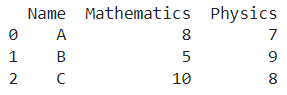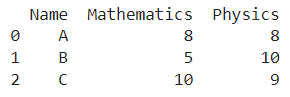# Update A Dataframe In Pandas While Iterating Row By Row

A DataFrame is the primary data structure of the Pandas library in Python and is commonly used for storing and working with tabular data.

A common operation that could be performed on such data is to update the DataFrame while iterating row by row to work with the information in a better manner.

To start working with Pandas, we first need to import it:

``import pandas as pd``

## Running Example

Let us understand this operation with the help of an example. Consider the following DataFrame containing 3 students with names A, B, and C and their corresponding marks (out of 10) for two subjects, Mathematics and Physics.Code snippet for generating the above DataFrame:

``````import pandas as pd

# Dictionary for our data
data = {'Name' : ['A', 'B', 'C'], 'Mathematics' : [8, 5, 10], 'Physics' : [7, 9, 8]}

# DataFrame for the dictionary
df = pd.DataFrame(data)

# Printing the DataFrame
print(df)``````

Here, data is a dictionary we created to initialize the DataFrame. For this, we use the DataFrame() function of the Pandas library which takes the dictionary as an argument and returns the required DataFrame.

Now, let’s say we need to update the contents of this dataframe while iterating row by row, for example, incrementing the contents of entries in the physics column. The resulting output would look like this :Let us look at different ways of performing this operation on a given DataFrame :

## 1. Update a Pandas Dataframe Using the values property

In this method, we use the .values property to access the values in a particular column. To access the physics column we use square brackets along with the label of the desired column.

After this, we use the values property along with the index of the required entry obtained from the for-loop. The for loop is run using the range function with the length, i.e the number of rows of the dataframe passed as a parameter. The updates are not made in place so reassignment is required.

Let us take a look at the corresponding code snippet and generated output for this method:

``````# Importing pandas
import pandas as pd

# Dictionary for our data
data = {'Name' : ['A', 'B', 'C'], 'Mathematics' : [8, 5, 10], 'Physics' : [7, 9, 8]}

# DataFrame for the dictionary
df = pd.DataFrame(data)

# Performing the operation
for i in range(len(df)):
df['Physics'].values[i] += 1

# Printing
print(df)``````

Output :Instead of using df[‘Physics’] to access the physics column, we could also simply use the dot operator in the form of df.Physics to get the same result. Let us take a look at the corresponding code snippet and generated output for this method:

``````# Importing pandas
import pandas as pd

# Dictionary for our data
data = {'Name' : ['A', 'B', 'C'], 'Mathematics' : [8, 5, 10], 'Physics' : [7, 9, 8]}

# DataFrame for the dictionary
df = pd.DataFrame(data)

# Performing the operation
for i in range(len(df)):
df.Physics.values[i] += 1

# Printing
print(df)``````

Output :## 2. Update A Dataframe Using list comprehension

In this method, we use the concept of a list comprehension to update the contents of the dataframe while iterating row by row. We use a for loop inside the list to populate it.

Square brackets are used to access the column of interest, here physics in the manner df[‘Physics’]. The updates are not made in place so reassignment is required.

Let us take a look at the corresponding code snippet and generated output for this method:

``````# Importing pandas
import pandas as pd

# Dictionary for our data
data = {'Name' : ['A', 'B', 'C'], 'Mathematics' : [8, 5, 10], 'Physics' : [7, 9, 8]}

# DataFrame for the dictionary
df = pd.DataFrame(data)

# Performing the operation
df['Physics'] = [x+1 for x in df['Physics']]

# Printing
print(df)``````

Output :Instead of using df[‘Physics’] to access the physics column, we could also simply use the dot operator in the form of df.Physics to get the same result. Let us take a look at the corresponding code snippet and generated output for this method:

``````# Importing pandas
import pandas as pd

# Dictionary for our data
data = {'Name' : ['A', 'B', 'C'], 'Mathematics' : [8, 5, 10], 'Physics' : [7, 9, 8]}

# DataFrame for the dictionary
df = pd.DataFrame(data)

# Performing the operation
df.Physics = [x+1 for x in df.Physics]

# Printing
print(df)``````

Output :## Conclusion

In this topic, we have learned to update an existing Pandas DataFrame while iterating row by row, following a running example of test scores of students in different subjects, thus giving us an intuition of how this concept could be applied in real-world situations. Feel free to reach out to [email protected] in case of any suggestions.

Trending